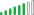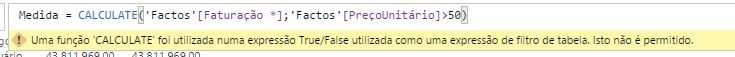cancel
Showing results for
Did you mean:Impactful Individual

## Using a Boolean expression with CALCULATE

Can someone explain me why this expression of a calculated measure works:

`Measure = CALCULATE(SUM(Facts[Turnover]);FILTER('Facts';'Facts'[UnitPrice]>=50))`

Whereas this one doesn't:

`Measure = CALCULATE(SUM(Facts[Turnover]);'Facts'[UnitPrice]>=50)`

I know CALCULATE can use a Boolean expression or a table expression that defines a filter, but isn't the second expression using a Bolean condition:

`'Facts'[UnitPrice]>=50`

1 ACCEPTED SOLUTIONSolution Supplier
This is correct. No measure or CALCULATE expression, unless you write a full FILTER expression around it.
9 REPLIES 9Community Champion

In what sense does the second formula "not work"? It appears to work fine in my test.

Proud to be a Super User!Impactful Individual

Hello,

I get the error:This means something like: "A CALCULATE function was used in a TRUE/FALSE expression as a table filter. This is not allowed".

And this message gets me even more confused.

However, the following function works perfectly:Community Champion

Are you adding that formula as a measure or a column?

Proud to be a Super User!Impactful Individual

As a measure.Solution Supplier

If 'Facts'[UnitPrice] is a calculated column, this expression

`Measure = CALCULATE(SUM(Facts[Turnover])                     ;'Facts'[UnitPrice]>=50)`

is just a shortcut for:

`Measure = CALCULATE(SUM(Facts[Turnover])                ;FILTER( ALL('Facts'[UnitPrice])                    ;'Facts'[UnitPrice]>=50                ))`

If 'Facts'[UnitPrice] is a measure, however, you will get an error message about CALCULATE not being allowed in a filter expression expression (of a CALCULATE expression).

Is it what is happening here?Impactful Individual

Yes, 'Facts'[UnitPrice] is a measure.Solution Supplier

Then you have your answer.Impactful Individual

Ok, so the answer is I can't use a calculated measure in CALCULATE but I can use in FILTER function, correct?Solution Supplier
This is correct. No measure or CALCULATE expression, unless you write a full FILTER expression around it.